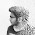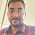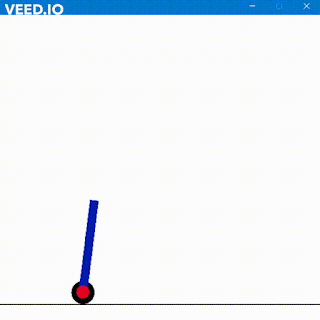## SEQUENCE DETECTOR

Beginning with the simple theory about Sequence Detector. A sequence detector an algorithm which detects a sequence within a given set of bits. Of course the length of total bits must be greater than sequence that has to be detected.
Sequence detector basically is of two types –

a.      Overlapping
b.     Non Overlapping
In overlapping some of the last bits can also be used for the start of detection of next sequence within the given bits.

For Example Let the sequence be 11011 and given bits 1101101101101101
Now lets work on overlapping concept.
We have 5 bits here in 11011 hence we will have 5 states. Let em be A/B/C/D and E.
Initially pstate will be A.
Now

1.     Incoming bit is 1 (from 1101101101101101) and it matches with first bit of sequence hence jump to next B. Requirement(1011)
2.     Incoming bit is 1 (from 1101101101101101)and it matches with  first bit of requirement hence jump to state C. Requirement (011)
3.     Incoming bit is 0 (from 1101101101101101) and it matches with first bit of requirement hence jump to state D. Requirement (11)
4.     Incoming bit is 1 (from 1101101101101101) and it matches with first bit of requirement hence jump to state E. Requirement (1)
5.     Incoming bit is 0 (from 1101101101101101) and it matches with first bit of requirement hence jump to state A. Requirement (). Output is 1 as we have found a sequence

To be more clear here is a table-

Notice that state C has 11 and requires 011. Now if it receives 1 instead of zero then instead of resetting and going back to A it will remain at C because C has 11 which can be used for starting of 11011 . This is called Overlapping. Similarly after state E we have restart to detect sequence then instead of starting again from A we will jump to C since it already has some bits which can serve as starting point. Remember always jump to that state which can provide maximum starting bits of sequence . Here B has 1 which can also serve but it isn’t maximum.

Ok again for better understanding we have 11011 then
11011  can serve as starting bit i.e state B
11011 can serve as starting bits i.e state C
11011 cannot serve as starting bits since sequence doesn’t start with 011
11011 cannot serve as starting bits since sequence doesn’t start with 1011

Here is the state diagram for this sequence. I am pretty sure you must have understood Overlapping till now. If No! you can contact or this state diagram should suffice.

Let's go step by step
(A) Idle state is A waiting for 1 which if it gets will jump to B else will remain to A

Now how many FFs do we require to make this machine. We have 5bits here so
by using this equation we can find
2x-1<5<2x
Thus we get X = 3 hence 3 FFs

To design in verilog here is the code for both Overlap and NonOverlap-

//***************************************************************************//
module sequence_detector(sequence,overlap,detect,clk,q);
input [15:0]sequence;
reg [15:0]temp;
reg bitin;
input overlap;
input [4:0]detect;
input clk;
output reg q;
integer i=0;
reg [2:0]pstate;
parameter A = 3'b000, B = 3'b001, C = 3'b011, D = 3'b100, E = 3'b101;
initial begin
pstate <=A;
\$monitor("Pstate=%d bit=%b q=%b",pstate,bitin,q);
end
always @(posedge clk)begin
if(i==0)
temp = sequence;
i = i + 1;
end
always @(posedge clk)begin
bitin = temp;
temp=temp<<1;
if(overlap==1'b1)begin
case(pstate)
A:
if(bitin==1'b1)begin
pstate = B;
q = 0;
end
else begin
pstate = A;
q = 0;
end
B:
if(bitin==1'b1)begin
pstate = C;
q = 0;
end
else begin
pstate = A;
q = 0;
end
C:
if(bitin==1'b0)begin
pstate = D;
q = 0;
end
else begin
pstate = C;
q = 0;
end
D:
if(bitin==1'b1)begin
pstate = E;
q = 0;
end
else begin
pstate = A;
q = 0;
end
E:
if(bitin==1'b1)begin
pstate = C;
q = 1;
end
else begin
pstate = A;
q = 0;
end
endcase
end
else if(overlap==1'b0) begin
case(pstate)
A:
if(bitin==1'b1)begin
pstate = B;
q = 0;
end
else begin
pstate = A;
q = 0;
end
B:
if(bitin==1'b1)begin
pstate = C;
q = 0;
end
else begin
pstate = A;
q = 0;
end
C:
if(bitin==1'b0)begin
pstate = D;
q = 0;
end
else begin
pstate = A;
q = 0;
end
D:
if(bitin==1'b1)begin
pstate = E;
q = 0;
end
else begin
pstate = A;
q = 0;
end
E:
if(bitin==1'b1)begin
pstate = A;
q = 1;
end
else begin
pstate = A;
q = 0;
end
endcase
end
end
endmodule

and here is the Test Bench
//*************************************************************************//
module sequence();
reg clk,overlap;
reg [4:0]detect;
reg [15:0]sequence;
wire q;

initial begin
clk = 0;
overlap = 1; //1 for overlap 0 for non overlap
sequence = 16'b1101101101101101;
detect = 5'b11011;
end
always #2 clk=!clk;
sequence_detector yeah(sequence,overlap,detect,clk,q);
endmodule
//**************************************************************************//

Here is the simulator output (Overlapping)

Here is the Console Output for Overlapping

Here is the simulator output (Non-Overlapping)

Here is the Console Output for NonOverlapping

Here is the State Table for this question

For the truth table here it is

D2 = X’*Y1 + X*Y2*Y0
D1 = X *Y0

D0 = X

Here is the data flow diagram

So Long

1.Nicely explained

1.2.This comment has been removed by the author.

1.Hey, Sorry your comment got deleted out accidentally My iPad isn't working well.
Well i is just a single step counter to store the data in temp first and then perform operations on it. We need to have a reserved copy to perform operation.
You can however do this in initial block also. It isn't compulsory to use i and an always block.

3.Can you please send the code for moore sequence detector for sequence-0111010

4.Can you please send the code for sequence -0111010

5.how to code non-overlapping?